﻿﻿ دسترسی همگانی(OPAC) نام کتابخانه در اوپک

### رکوردهای مرتبط با این نویسنده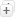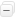مرتب سازی     درج پیشنهاد خرید   پالایش جستجو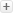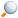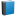Mathematical handbook of formulas and tables (1968) / Spiegel ، Murray R، نویسنده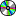Real variables (1969) / Spiegel ، Murray R، نویسندهSchaum's outline of theory and problems of advanced calculus (1963) / Spiegel ، Murray R، نویسندهSchaum's outline of theory and problems of advanced mathematics for engineers and scientists (1971) / Spiegel ، Murray R، نویسندهSchaum's outline of theory and problems of vector analysis and an introduction to tensor analysis (1959) / Spiegel ، Murray R، نویسندهSchaum`s outline of theory and problems of fourier analysis with applications to boundary value problems (1974) / Spiegel ، Murray R، نویسندهSchaum’s outline of theory and problems of advanced calculus (c2002) / Wrede ، Robert C، نویسندهTheory and problems of probability and statistics (1975) / Spiegel ، Murray R، نویسنده